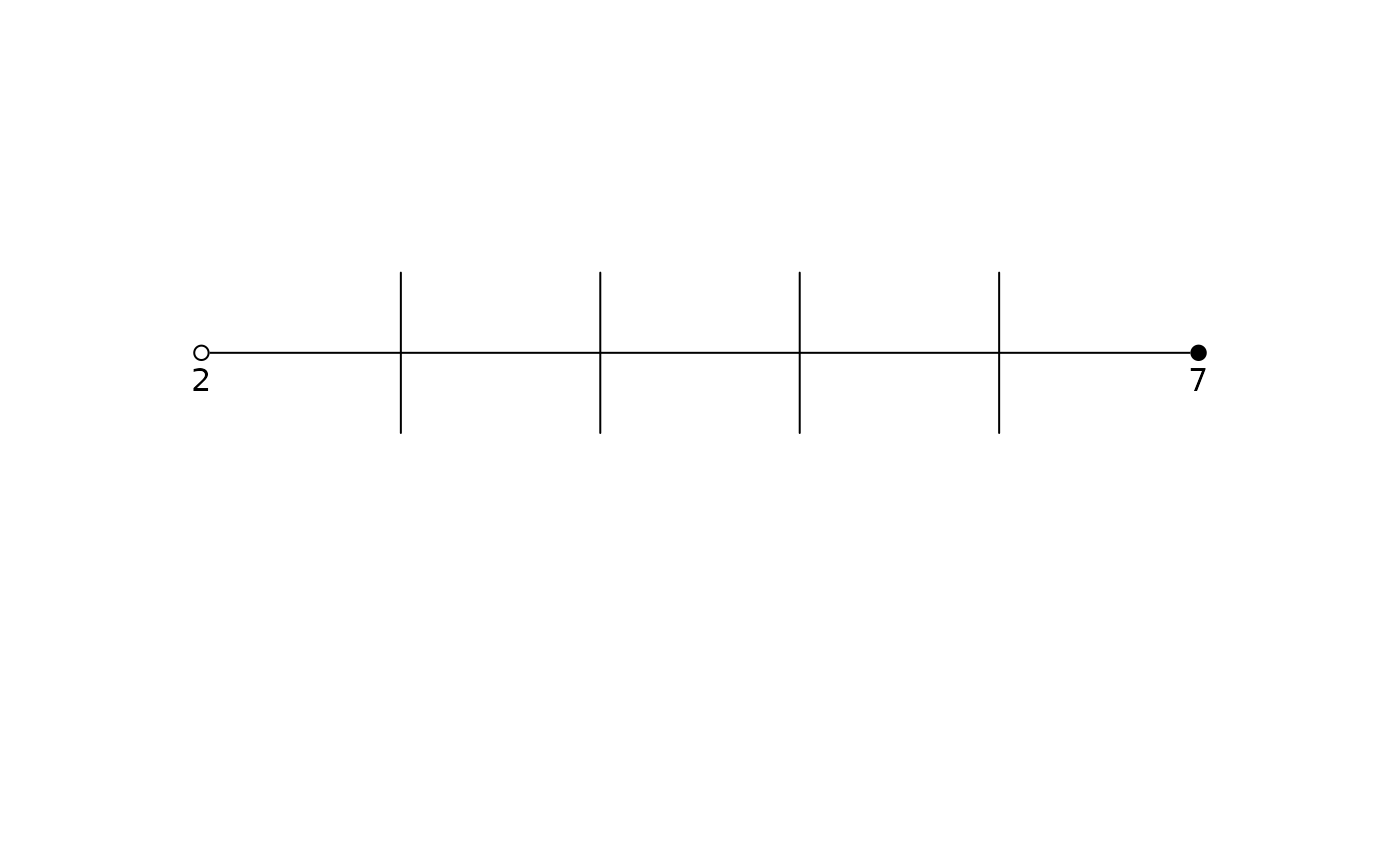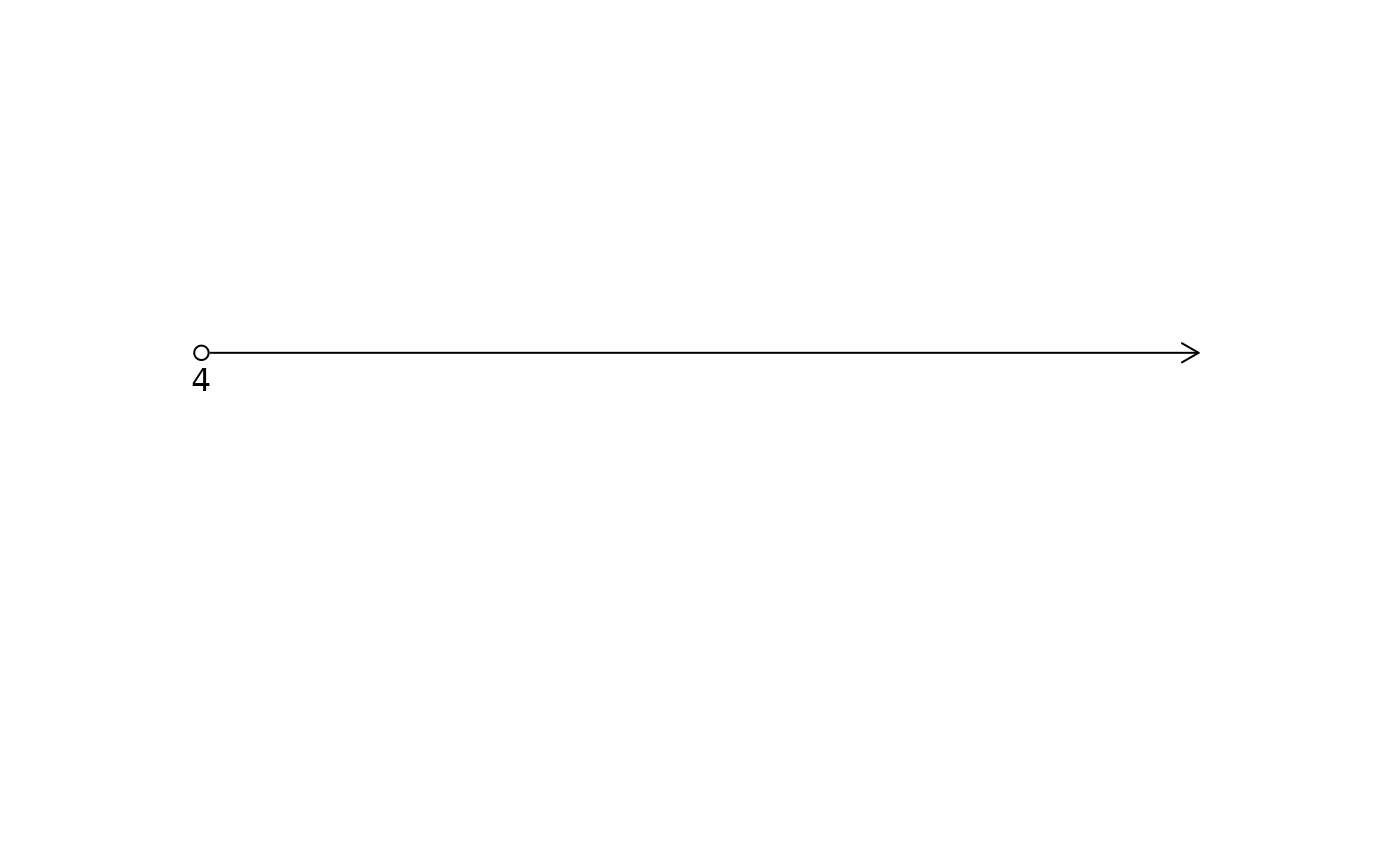Creae a simple plot showing a line segment.

## Usage

lsegments(
x = c(3, 7),
l = "o",
r = "c",
ticks = TRUE,
labs = 1,
ylim = c(-0.75, 0.25)
)

## Arguments

x

The endpoints of the interval. Values larger (smaller) than 999 (-999) will be interpreted as (negative) infinity.

l

Indicate whether the left end point should be open ("o") or closed ("c").

r

Indicate whether the right end point should be open ("o") or closed ("c").

ticks

Indicate whether to show tick marks (TRUE) or not (FALSE).

labs

The position for the point labels. Set to 0 if no labels should be shown.

Indicate whether the line segment should be added to an existing plot (TRUE) or a new plot should be created (FALSE).

ylim

A vector of length 2 specifying the vertical plotting limits, which may be useful for fine-tuning plots. The default is c(-0.75,0.25).

dlsegments, CCP, ArrowLines

David Diez

## Examples


lsegments(c(2, 7), "o", "c", ylim = c(-0.3, 0.2))lsegments(c(5, 7), "c", "c", ylim = c(-0.3, 0.2))lsegments(c(4, 1000), "o", "o", ylim = c(-0.3, 0.2))